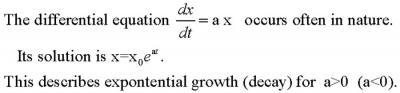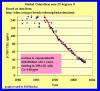|

# Graphs of Exponential Growth/DecayHave your students analyze real data that fit exponential decay to estimate the residence time of a system. The residence time is the time it takes for the level to drop to 37% (1/e) its initial value after all inflow has stopped.
Examples of data that fit exponential decay are: Radioactive decay, first order loss rates in the atmosphere (click figure on left below for an example), lakes, ocean, solid earth, or biosphere, and river gage level after a significant rainfall event (click figure on right below for an example). A good analogy is water loss from a bucket with a hole in the bottom. See Mass Balance with Water Bucket Model for an example of this.More USGS River Data
By graphing the natural log vs time the exponential decay graph becomes linear. This makes it easier to obtain a more precise estimate of the residence time.
Although exponential growth is always ultimately limited it is a good approximation to many physical processes in the Earth system for finite time intervals. Examples include: population growth, economic growth, growth of carbon emissions,